Beams Index

Shear stresses /deflections in Beams in Bending

Introduction

A normally loaded beam is subject to both bending and shear forces.    The standard equations for stress and strain for beams ( flexure formulae ) generally only consider the bending stresses and strains. The shear stresses and strains are not considered as they are generally small relative to the bending stresses and deflections.  For normal engineering applications the bending equations are valid if shear forces are present or not.   The notes below relate to the evaluation of shear stresses and resultant deflections present in a beam subject to bending and shear forces.

Nomenclature
 A= Section area (m2) A'= Section area of beam between y1 and outer surface(m2) e = strain σ = direct stress (N/m2) τ = shear stress (N/m2) E = Young's Modulus = σ /e (N/m2) y = distance of surface from neutral surface (m). R = Radius of neutral axis (m). I = Moment of Inertia (m4 - more normally cm4) Z = section modulus = I/ymax(m3 - more normally cm3) M = Moment (Nm) w = Distributed load on beam (kg/m) or (N/m as force units) W = total load on beam (kg ) or (N as force units) F= Concentrated force on beam (N) S = Shear Force on Section (N) L = length of beam (m) x = distance along beam (m) Fa, Fb,Fc Force in horizontal direction. ( N ) Q = First Moment of area of A' about neutral axis of section(m3)

Shear stresses

Consider a portion of a beam subject to a moment and a shear force. The beam is assumed to be of rectangular section of uniform width b.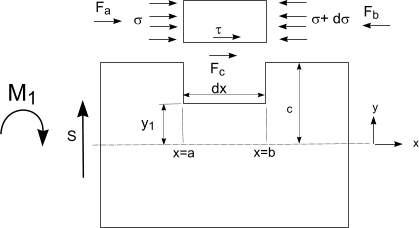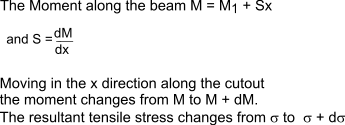To get the the horizontal forces at a and b.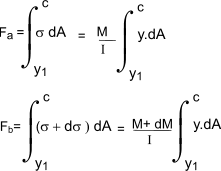Calculating the resultant shear force and stress.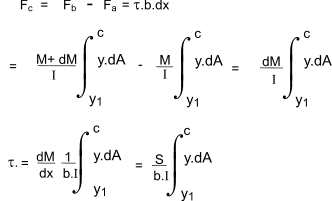The integral is actually the first moment of area of the vertical face above y1 abut the section neutral axis.   For these notes this moment is designated Q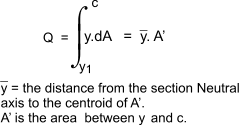Therefore based on the specific definitions of Q and b relative to y1 the shear stress at any value of y1 is given by .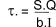Now considering the stress distribution in a rectangular beam subject to shear loading and bending.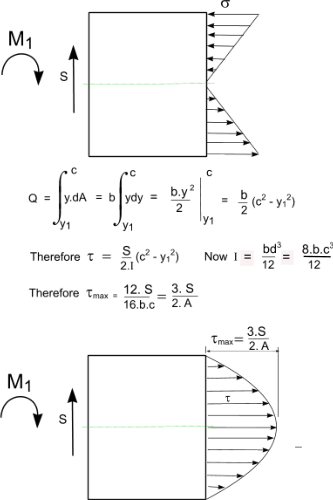It is clear from the above notes that for a beam subject to shear loading and bending the maximum shear stress is at the neutral axis and reduces to zero at the the outer surfaces wher y1 = ± c .   For this section the maximum stress is equal to 3/2 the average shear stress = S / A .

The maximum shear stress in other sections are shown below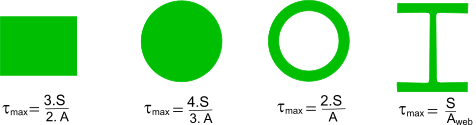The notes above relate to plane stress and the following figure extracted from my notes on Mohr's circle Mohr's circle illustrate that, to maintain equilibrium, the horizontal shear stress i.e τ yx is equal to the vertical shear stress i.e .τ xyat the same point.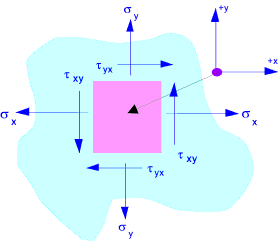Example. Showing how the shear stress can have an impact on a bending moment calculation is provided below..The maximum bending stress occurs at x = 100mm.
The effect of the shear stress is maximised at y1 = 45mm. The section under consideration is a hollow square section 100mm square with wall thickness = 5mm. Note the corner radii are ignored to simplify the calculation.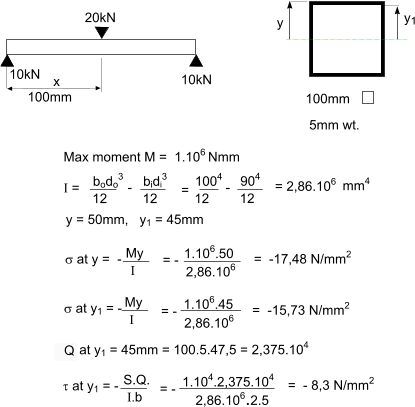The notes provided on the above identified Mohr's circle webpage show how to determine the principal stresses σ 1 and σ 2 from the direct and shear stresses at a point.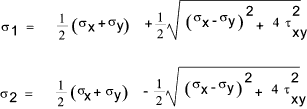Applying these equations to obtain the relevant principal stresses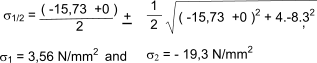It is clear from this example that the shear stresses can under some circumstances affect the design stress for a beam..  In most cases however the effect is minimal..

Deflection of beams due to shear

The notes below relate primarily to rectanugular section beams.

Determination of deflection of beams using strain energy methods.

When a material is subject to shear strain, within the elastic limit the elastic strain energy is stored just as in the case of direct strain energy.

Consider an section of material of width W perpendicular to the section..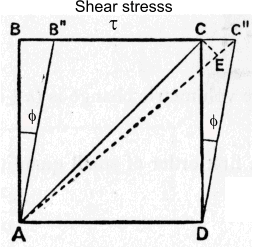The uniform shear stress on the top surface is τ resulting in a shear strain of φ and a deflection of BB". The stored strain energy =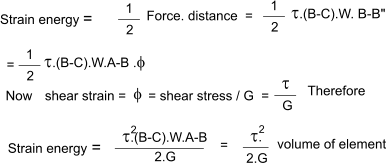The above simple equation is the strain energy stored in an element .
The strain energy stored in the whole beam is expresed as.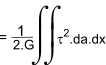Now consider a beam of length L with an end Vertical force of W.    This force is uniform along the whole length of the beam.     If the shear force was evenly distributed over the whole section the end deflection due to shear would be L x (angle of shear).

deflection = L. φ = L.(τ/G ) or W.L /A,G

The shear force is, however not distributed over the whole section.    Assuming the various equations above for the distribution of shear stress across a vertical section are correct for a rectangular beam the following derivation of the shear deflection for a cantilever beam is provided.

Consider a beam with section width b, and depth d.A strip of this beam of longitudonal of length L , width b and depth dy parallel to the neutral axis (NA)_and distance y from the (NA) will store strain energy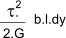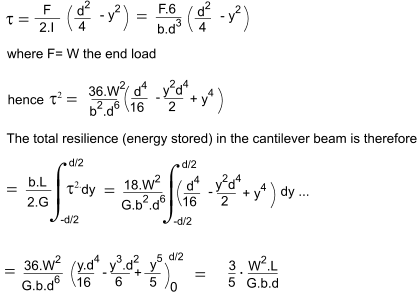Now consider that the deflection at the free end of the cantilever beam = δ. The shear energy stored =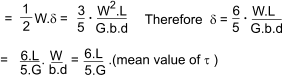The deflections due to shear stress for other loading scenarios are shown below

Rectangular section beams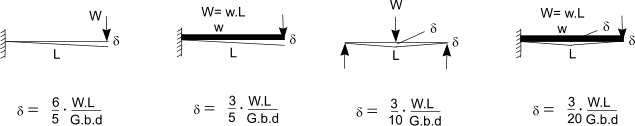I section beams.
b and d relate to web dimensions of beam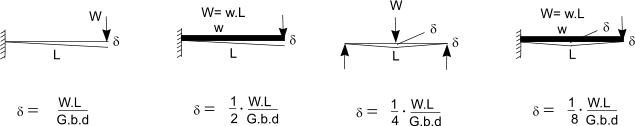Sites Providing Relevant Information Deflection Coefficients..Notes clarifying deflection equations provided above Shear stresses in beams.. concise paper with clear useful information Lecture:beams shearing stress.. Extremely clear pdf download

Beams Index